# Solution assignment 20 Quadratic equations (factorizing)

### Assignment 20

Try to solve by factorizing: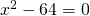### Solution

In this case we can factorize the function quite easily, by applying one of the special products: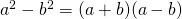Because 64 is the square of 8, we can write the equation as: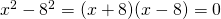and thus the solutions are: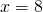or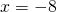0